Specialists in Linear Flow Analysis

# SC10 2D Compressor profile

Standard Configuration 10 is an excellent test case, due to the quality, quantity and availability of numerical solutions from other methods. It is an Euler test case. Many inviscid features (correct Euler formulation, correct far-field boundary treatment, and correct treatment of shocks) of a numerical method can be tested via this test case. The solutions from the other methods (Verdon, Hall and Huff) shown here can be found at the Standard Configuration 10 home page.

# Geometry

Standard configuration 10 is a two-dimensional compressor cascade. The profile is constructed by superimposing the thickness distribution of a modified NACA0006 airfoil on a circular-arc camber line. The thickness distribution is given by:
t(s) = 0.06 × (2.969 s½ - 1.26 s - 3.516 s2 + 2.843 s3 - 1.036 s4),   0 <= s <= 1.

Note that the modification gives zero thickness at the trailing edge, that is, a wedge shaped edge. The camber distribution is given by:
c(s) = -2.475 + [ r2 - (s - 0.5)2]½, 0 <= s <= 1, where the radius of the camber, r = 2.525.

The upper surface of the profile is given by
x = s - 0.5 t(s) sin(Ø)
y = c(s) + 0.5 t(s) cos(Ø)
and the lower surface of the profile is given by
x = s + 0.5 t(s) sin(Ø)
y = c(s) - 0.5 t(s) cos(Ø)
where Ø is the tan-1 (dC/ds).

The stagger angle is 45 degrees and the pitch to chord ratio is 1.0.

Two steady-state flow conditions have been defined for Standard Configuration 10. The inlet Mach Numbers (M1), inlet flow angles (α1), 2D flow-field plots, surface pressure coefficient plots, surface isentropic Mach number plots, and the raw RPMTurbo data for the two conditions are shown in the table below.

Case M1 α1 Flow-Field Plot Cp Plot
Isentropic Mach
Number Plot
RPMTurbo Solution
Subsonic 0.7 55.0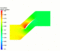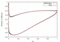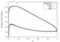[Data]
Transonic 0.8 58.0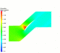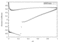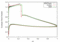[Data]

A total of 32 different unsteady test cases have been defined for Standard Configuration 10. Selected test cases are shown in the table below. Two mode shapes are examined: pitching about (0.5, 0.05) in profile co-ordinates, and bending normal to the chord. In the table below, ω* is the reduced frequency based on full chord and σ is the interblade phase-angle.

Coefficient Plot
RPMTurbo Solution
1 subsonic pitching 0.5 0.0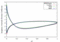[Data]
2 subsonic pitching 0.5 90.0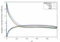[Data]
6 subsonic pitching 1.5 90.0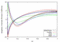[Data]
9 subsonic bending 0.5 0.0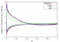[Data]
17 transonic pitching 0.5 0.0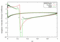[Data]

# Aerodynamic Damping versus Interblade Phase-Angle

Steady Case Mode Shape ω* Plot Data
subsonic pitching 0.5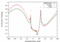[Data]
subsonic pitching 1.0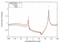[Data]
subsonic bending 0.5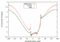[Data]
transonic pitching 0.5[Data]